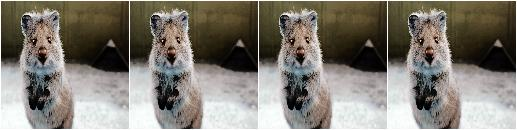# augmenters.contrast¶

## GammaContrast¶

Adjust image contrast by scaling pixel values to `255*((v/255)**gamma)`.

Values in the range `gamma=(0.5, 2.0)` seem to be sensible.

API link: `GammaContrast()`

Example. Modify the contrast of images according to `255*((v/255)**gamma)`, where `v` is a pixel value and `gamma` is sampled uniformly from the interval `[0.5, 2.0]` (once per image):

```import imgaug.augmenters as iaa
aug = iaa.GammaContrast((0.5, 2.0))
```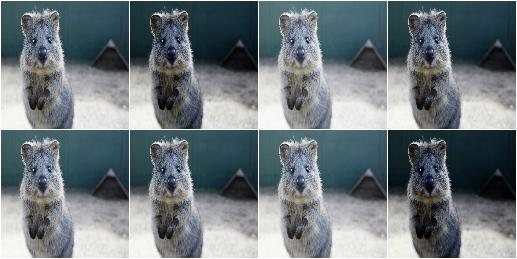Example. Same as in the previous example, but `gamma` is sampled once per image and channel:

```aug = iaa.GammaContrast((0.5, 2.0), per_channel=True)
```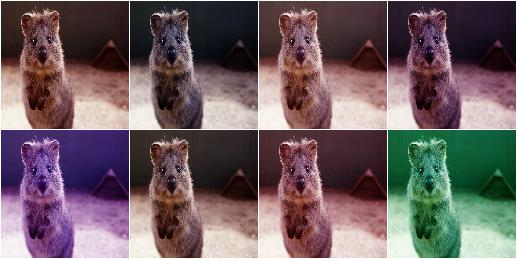## SigmoidContrast¶

Adjust image contrast to `255*1/(1+exp(gain*(cutoff-I_ij/255)))`.

Values in the range `gain=(5, 20)` and `cutoff=(0.25, 0.75)` seem to be sensible.

API link: `SigmoidContrast()`

Example. Modify the contrast of images according to `255*1/(1+exp(gain*(cutoff-v/255)))`, where `v` is a pixel value, `gain` is sampled uniformly from the interval `[3, 10]` (once per image) and `cutoff` is sampled uniformly from the interval `[0.4, 0.6]` (also once per image).

```import imgaug.augmenters as iaa
aug = iaa.SigmoidContrast(gain=(3, 10), cutoff=(0.4, 0.6))
```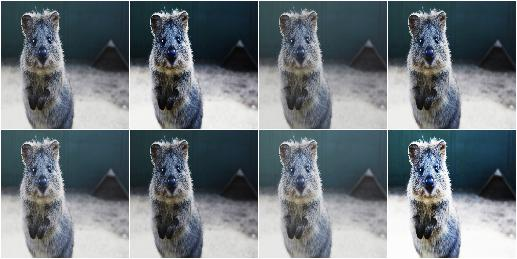Example. Same as in the previous example, but `gain` and `cutoff` are each sampled once per image and channel:

```aug = iaa.SigmoidContrast(
gain=(3, 10), cutoff=(0.4, 0.6), per_channel=True)
```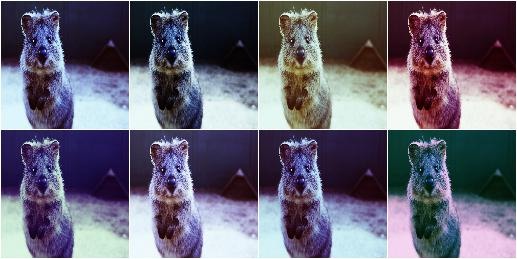## LogContrast¶

Adjust image contrast by scaling pixels to `255*gain*log_2(1+v/255)`.

This augmenter is fairly similar to `imgaug.augmenters.arithmetic.Multiply`.

API link: `LogContrast()`

Example. Modify the contrast of images according to `255*gain*log_2(1+v/255)`, where `v` is a pixel value and `gain` is sampled uniformly from the interval `[0.6, 1.4]` (once per image):

```import imgaug.augmenters as iaa
aug = iaa.LogContrast(gain=(0.6, 1.4))
```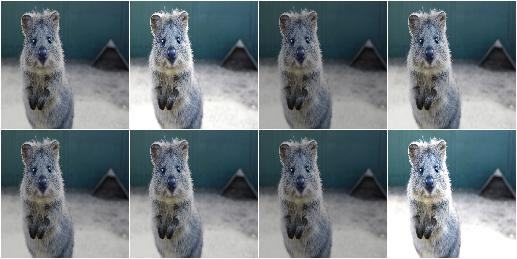Example. Same as in the previous example, but `gain` is sampled once per image and channel:

```aug = iaa.LogContrast(gain=(0.6, 1.4), per_channel=True)
```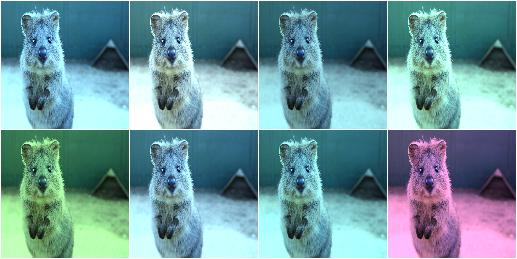## LinearContrast¶

Adjust contrast by scaling each pixel to `127 + alpha*(v-127)`.

API link: `LinearContrast()`

Example. Modify the contrast of images according to 127 + alpha*(v-127)`, where `v` is a pixel value and `alpha` is sampled uniformly from the interval `[0.4, 1.6]` (once per image):

```import imgaug.augmenters as iaa
aug = iaa.LinearContrast((0.4, 1.6))
```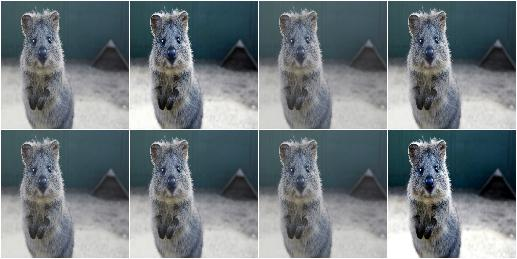Example. Same as in the previous example, but `alpha` is sampled once per image and channel:

```aug = iaa.LinearContrast((0.4, 1.6), per_channel=True)
```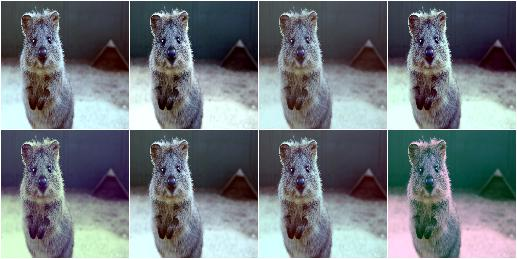## AllChannelsCLAHE¶

Apply CLAHE to all channels of images in their original colorspaces.

CLAHE (Contrast Limited Adaptive Histogram Equalization) performs histogram equilization within image patches, i.e. over local neighbourhoods.

In contrast to `imgaug.augmenters.contrast.CLAHE`, this augmenter operates directly on all channels of the input images. It does not perform any colorspace transformations and does not focus on specific channels (e.g. `L` in `Lab` colorspace).

API link: `AllChannelsCLAHE`

Example. Create an augmenter that applies CLAHE to all channels of input images:

```import imgaug.augmenters as iaa
aug = iaa.AllChannelsCLAHE()
```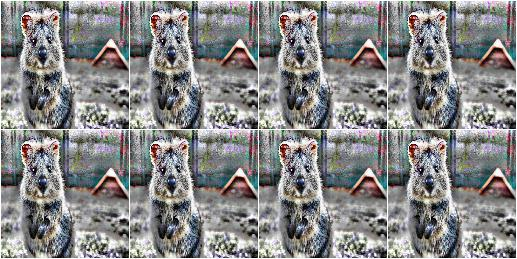Example. Same as in the previous example, but the clip_limit used by CLAHE is uniformly sampled per image from the interval `[1, 10]`. Some images will therefore have stronger contrast than others (i.e. higher clip limit values).

```aug = iaa.AllChannelsCLAHE(clip_limit=(1, 10))
```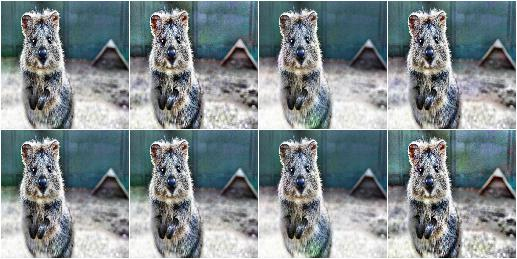Example. Same as in the previous example, but the clip_limit is sampled per image and channel, leading to different levels of contrast for each channel:

```aug = iaa.AllChannelsCLAHE(clip_limit=(1, 10), per_channel=True)
```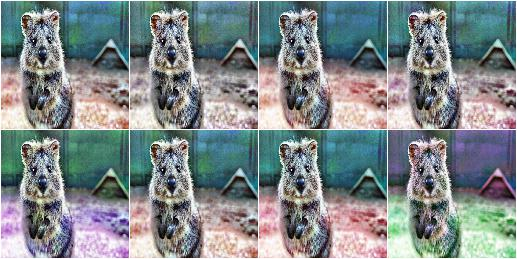## CLAHE¶

Apply CLAHE to L/V/L channels in HLS/HSV/Lab colorspaces.

This augmenter applies CLAHE (Contrast Limited Adaptive Histogram Equalization) to images, a form of histogram equalization that normalizes within local image patches. The augmenter transforms input images to a target colorspace (e.g. `Lab`), extracts an intensity-related channel from the converted images (e.g. `L` for `Lab`), applies CLAHE to the channel and then converts the resulting image back to the original colorspace.

Grayscale images (images without channel axis or with only one channel axis) are automatically handled, from_colorspace does not have to be adjusted for them. For images with four channels (e.g. `RGBA`), the fourth channel is ignored in the colorspace conversion (e.g. from an `RGBA` image, only the `RGB` part is converted, normalized, converted back and concatenated with the input `A` channel). Images with unusual channel numbers (2, 5 or more than 5) are normalized channel-by-channel (same behaviour as `AllChannelsCLAHE`, though a warning will be raised).

If you want to apply CLAHE to each channel of the original input image’s colorspace (without any colorspace conversion), use `imgaug.augmenters.contrast.AllChannelsCLAHE` instead.

API link: `CLAHE`

Example. Create a standard CLAHE augmenter:

```import imgaug.augmenters as iaa
aug = iaa.CLAHE()
```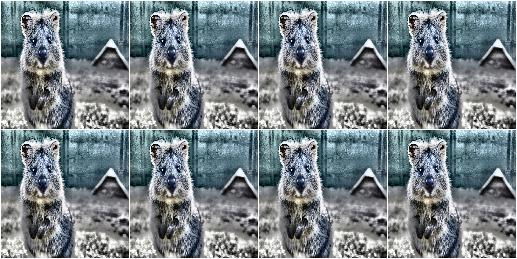Example. Create a CLAHE augmenter with a clip limit uniformly sampled from `[1..10]`, where `1` is rather low contrast and `10` is rather high contrast:

```aug = iaa.CLAHE(clip_limit=(1, 10))
```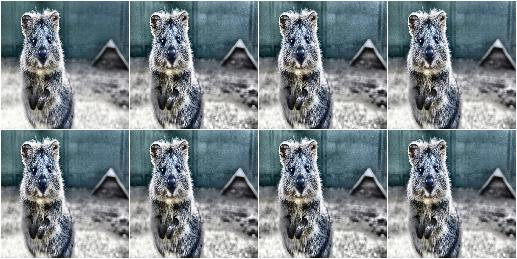Example. Create a CLAHE augmenter with kernel sizes of `SxS`, where `S` is uniformly sampled from `[3..21]`. Sampling happens once per image.

```aug = iaa.CLAHE(tile_grid_size_px=(3, 21))
```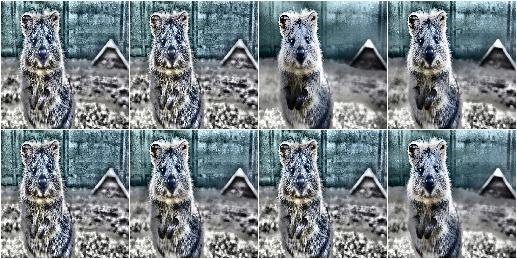Example. Create a CLAHE augmenter with kernel sizes of `SxS`, where `S` is sampled from `N(7, 2)`, but does not go below `3`:

```import imgaug.parameters as iap
aug = iaa.CLAHE(
tile_grid_size_px=iap.Discretize(iap.Normal(loc=7, scale=2)),
tile_grid_size_px_min=3)
```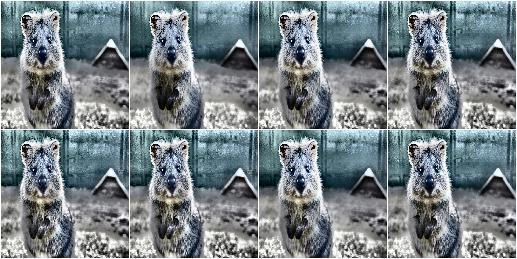Example. Create a CLAHE augmenter with kernel sizes of `HxW`, where `H` is uniformly sampled from `[3..21]` and `W` is randomly picked from the list `[3, 5, 7]`:

```aug = iaa.CLAHE(tile_grid_size_px=((3, 21), [3, 5, 7]))
```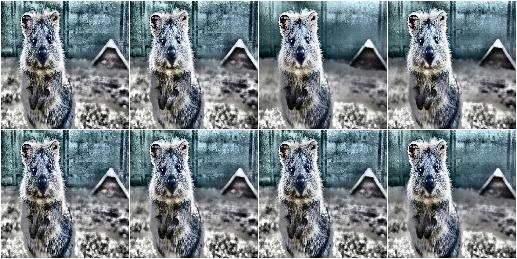Example. Create a CLAHE augmenter that converts images from BGR colorspace to HSV colorspace and then applies the local histogram equalization to the `V` channel of the images (before converting back to `BGR`). Alternatively, `Lab` (default) or `HLS` can be used as the target colorspace. Grayscale images (no channels / one channel) are never converted and are instead directly normalized (i.e. from_colorspace does not have to be changed for them).

```aug = iaa.CLAHE(
from_colorspace=iaa.CLAHE.BGR,
to_colorspace=iaa.CLAHE.HSV)
```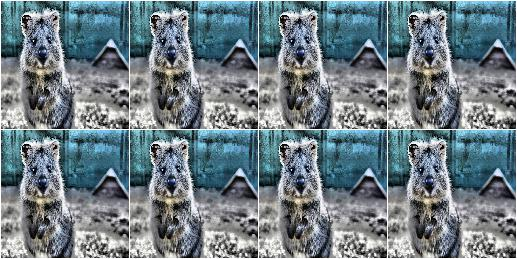## AllChannelsHistogramEqualization¶

Apply Histogram Eq. to all channels of images in their original colorspaces.

In contrast to `imgaug.augmenters.contrast.HistogramEqualization`, this augmenter operates directly on all channels of the input images. It does not perform any colorspace transformations and does not focus on specific channels (e.g. `L` in `Lab` colorspace).

Example. Create an augmenter that applies histogram equalization to all channels of input images in the original colorspaces:

```import imgaug.augmenters as iaa
aug = iaa.AllChannelsHistogramEqualization()
```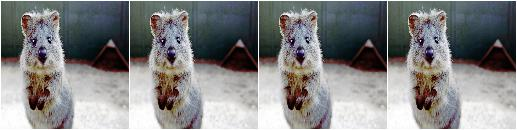Example. Same as in the previous example, but alpha-blends the contrast-enhanced augmented images with the original input images using random blend strengths. This leads to random strengths of the contrast adjustment.

```aug = iaa.Alpha((0.0, 1.0), iaa.AllChannelsHistogramEqualization())
```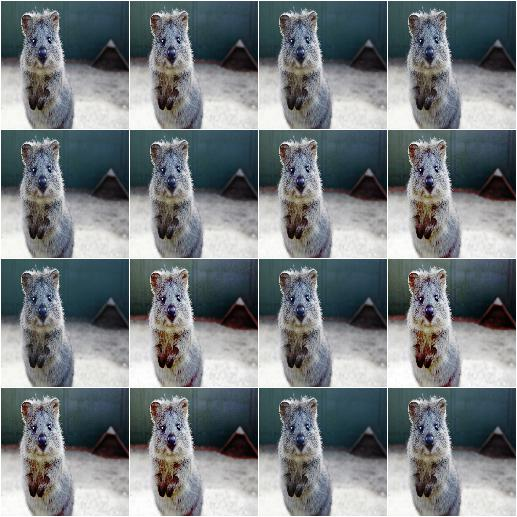## HistogramEqualization¶

Apply Histogram Eq. to L/V/L channels of images in HLS/HSV/Lab colorspaces.

This augmenter is similar to `imgaug.augmenters.contrast.CLAHE`.

The augmenter transforms input images to a target colorspace (e.g. `Lab`), extracts an intensity-related channel from the converted images (e.g. `L` for `Lab`), applies Histogram Equalization to the channel and then converts the resulting image back to the original colorspace.

Grayscale images (images without channel axis or with only one channel axis) are automatically handled, from_colorspace does not have to be adjusted for them. For images with four channels (e.g. RGBA), the fourth channel is ignored in the colorspace conversion (e.g. from an `RGBA` image, only the `RGB` part is converted, normalized, converted back and concatenated with the input `A` channel). Images with unusual channel numbers (2, 5 or more than 5) are normalized channel-by-channel (same behaviour as `AllChannelsHistogramEqualization`, though a warning will be raised).

If you want to apply HistogramEqualization to each channel of the original input image’s colorspace (without any colorspace conversion), use `imgaug.augmenters.contrast.AllChannelsHistogramEqualization` instead.

API link: `HistogramEqualization`

Example. Create an augmenter that converts images to `HLS`/`HSV`/`Lab` colorspaces, extracts intensity-related channels (i.e. `L`/`V`/`L`), applies histogram equalization to these channels and converts back to the input colorspace:

```import imgaug.augmenters as iaa
aug = iaa.HistogramEqualization()
```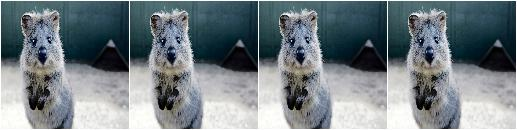Example. Same as in the previous example, but alpha blends the result, leading to various strengths of contrast normalization:

```aug = iaa.Alpha((0.0, 1.0), iaa.HistogramEqualization())
```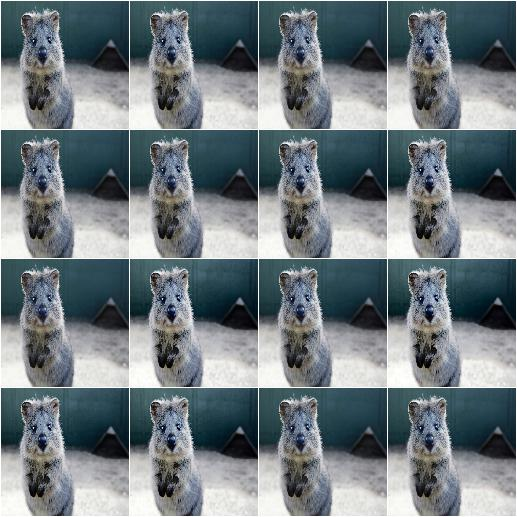Example. Same as in the first example, but the colorspace of input images has to be `BGR` (instead of default `RGB`) and the histogram equalization is applied to the `V` channel in `HSV` colorspace:

```aug = iaa.HistogramEqualization(
from_colorspace=iaa.HistogramEqualization.BGR,
to_colorspace=iaa.HistogramEqualization.HSV)
```